Courses

# Test Level 2: Units And Dimensions- 2

## 11 Questions MCQ Test Physics Class 11 | Test Level 2: Units And Dimensions- 2

Description
This mock test of Test Level 2: Units And Dimensions- 2 for Class 11 helps you for every Class 11 entrance exam. This contains 11 Multiple Choice Questions for Class 11 Test Level 2: Units And Dimensions- 2 (mcq) to study with solutions a complete question bank. The solved questions answers in this Test Level 2: Units And Dimensions- 2 quiz give you a good mix of easy questions and tough questions. Class 11 students definitely take this Test Level 2: Units And Dimensions- 2 exercise for a better result in the exam. You can find other Test Level 2: Units And Dimensions- 2 extra questions, long questions & short questions for Class 11 on EduRev as well by searching above.
QUESTION: 1

### Identify the pair whose dimensions are equal. [AIEEE 2002]

Solution:

The dimensions of torque and work are [ML2T-2]

QUESTION: 2

### The physical quantities not having same dimensions are [AIEEE 2003]

Solution:

Planck's constant (in term of unit)
(h) = j-s
= [ML2T-2][T]
= [ML2T-1]
Momentum (p) = kg-ms-1
=[M][L][T-1] = [MLT-1]

QUESTION: 3

### Dimensions of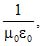where symbols have their usual meaning, are [AIEEE 2003]

Solution:

As we know that formula of velocity is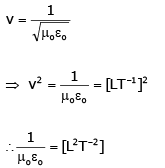QUESTION: 4

Which one of the following represents the correct dimensions of the coefficient of viscosity?

[AIEEE 2004]

Solution:

By Newton's formula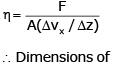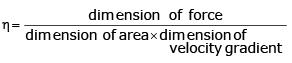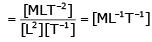QUESTION: 5

Out of the following pairs, which one does not have identical dimensions ?

[AIEEE 2005]

Solution:

I = mr2
∴[I] = [ML2]
and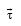= moment of force =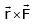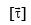= [L][MT-2]=[ML2T-2]

QUESTION: 6

The 'rad' is the correct unit used to report the measurement of

[AIEEE 2006]

Solution:

QUESTION: 7

Which of the following units denotes the dimensions [ML2/Q2], where Q denotes the electric charges ?

[AIEEE 2006]

Solution:

Magnetic energy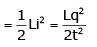[L→ inductance, i → current]
Energy has the dimension = [ML2T-2]
Equate the dimension, we have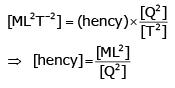QUESTION: 8

A body of mass m = 3.513 kg is moving along the x-axis with a speed of 5.00 ms-1. The magnitude of its momentum is recorded as

[AIEEE 2008]

Solution:

m = 3.513 kg and v = 5.00 ms-1
So momentum, p = mv = 17.565 As the number of significant digits in m is 4 and in v is 3, so p must have 3 (minimum) significant digits.
Hence, p = 17.6 kg ms-1

QUESTION: 9

The dimensions of magnetic field in M, L, T and C (coulomb) is given as

[AIEEE 2008]

Solution: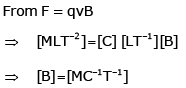QUESTION: 10

In an experiment the angles are required to be measured using an instrument. 29 divisions of the main scale exactly coincide with the 30 divisions of the vernier scale. If the smallest division of the main scale is half-a-degree (= 0.5°), then the least count of the instrument is

[AIEEE 2009]

Solution: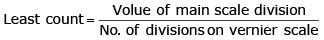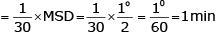QUESTION: 11

The respective number of significant figures for the numbers 23.023, 0.0003 and 2.1 × 10–3 are

Solution:

(i) All the non-zero digits are significant.

(ii) All the zeros between two non-zero digits are significant, no matter where the decimal point is, if at all.

(iii) If the number is less than 1, the zero(s) on the right of decimal point but to the left of the first non-zero digit are not significant.

(iv) The power of 10 is irrelevant to the determination of significant figures. According to the above rules, 23.023 has 5 significant figures. 0.0003 has 1 significant figures. 2.1 × 10–3 has 2 significant figures.